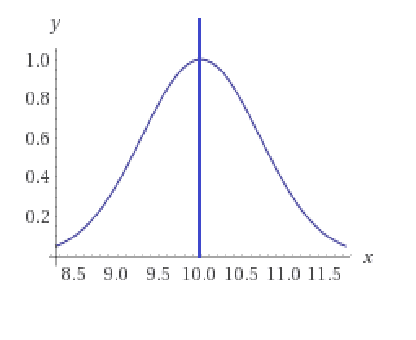# If 0.999... = 1 , Does 0.00...1 = 0

• B
Sorry if the question has been asked before, but is there any proof that 0.00...1 equals 0?
Or not, as the case may be
Thanks for any replies :)

fresh_42
Mentor
What is
0.00...1
? Where is the one placed at?

•cyclogon
mfb
Mentor
0.00...1 is not a well-defined expression.

0.00... = 0.

•cyclogon
fresh_42
Mentor
Here's a more interesting example. Consider the function ##x \longmapsto e^{-(x-n)^2}##. For ##n=10## it looks likeand the greater ##n## is, the more to the right is the bump. The area below the curve is ##\int_{-\infty}^{+\infty} e^{-(x-n)^2}\, dx \,=\, \sqrt{\pi}\,##. If we move it to infinity, then it is still the same bump with the same area ##\lim_{n \to \infty} \int_{-\infty}^{+\infty} e^{-(x-n)^2}\, dx \,=\, \sqrt{\pi}##. However, if we first move the bump to infinity, we get ## \lim_{n \to \infty} e^{-(x-n)^2} = 0## and the area ##\int_{-\infty}^{+\infty} (\lim_{n \to \infty} e^{-(x-n)^2})\,dx ## vanishes with it. This is the same with your ##1##. If we move it to infinity, it vanishes, because wherever we look, we are left from the bump and arbitrary close to zero, the bump is never reached.

#### Attachments

•sysprog, cyclogon and QuantumQuest
Mark44
Mentor
There's a huge difference between 0.999... and 0.000...1 that is alluded to in post #2. In the first expression, the ellipsis (...) means that the same pattern of 9 digits repeats endlessly, so that each position to the right of the decimal point contains the digit 9. In the other expression, it's not specified where that 1 digit is, making it not well-defined.

•cyclogon
Perhaps better written as $$\lim_{n\to \infty} \frac{1}{10^n}=0$$

But keep in mind that is the limit. And that is different than zero.

•cyclogon
hi, thanks for all your replies. Sorry about not explaining clearing enough.
The "1" is at the end of a length of infinitely many zeros
ie. 0.0000000...(infinte zeros)....1

Thanks

phinds
Gold Member
hi, thanks for all your replies. Sorry about not explaining clearing enough.
The "1" is at the end of a length of infinitely many zeros
ie. 0.0000000...(infinte zeros)....1
Yes, I think people probably realized that's what you meant, but there is no such thing which is why folks are saying that it's undefined. If you get to a point where you can put a 1, then you are not yet at infinity so your statement is nonsensical/undefined.

•cyclogon
russ_watters
Mentor
The "1" is at the end of a length of infinitely many zeros
ie. 0.0000000...(infinte zeros)....1
This is a self-contradiction: if the string of zeros is infinite, it doesn't have an end. That's why that expression doesn't work isn't used in math.

•cyclogon
jbriggs444
Homework Helper
Perhaps better written as $$\lim_{n\to \infty} \frac{1}{10^n}=0$$

But keep in mind that is the limit. And that is different than zero.
That limit is not different from zero. It is precisely zero. As one can see from the epsilon/delta definition of a limit.

•cyclogon and phinds
jbriggs444
Homework Helper
This is a self-contradiction: if the string of zeros is infinite, it doesn't have an end. That's why that expression doesn't work isn't used in math.
To be picky, one could index the digits in a decimal string over a set of positions with order type omega plus one. The difficulty is not that this is a self-contradiction. The difficulty is that the resulting digit strings do not naturally form an algebraic field.

•cyclogon and fresh_42
That limit is not different from zero. It is precisely zero. As one can see from the epsilon/delta definition of a limit.

Perhaps I should have said the limit is zero but the sequence 1/10, 1/100, ... is never precisely zero.

•cyclogon
Mark44
Mentor
Perhaps I should have said the limit is zero but the sequence 1/10, 1/100, ... is never precisely zero.
The sequence, which is just a list of numbers, is never precisely anything. This sequence converges to zero, although no element of the sequence is zero.

•cyclogon and fresh_42
jbriggs444
Homework Helper
Perhaps I should have said the limit is zero but the sequence 1/10, 1/100, ... is never precisely zero.
I would phrase it that "no term of the sequence is zero".

Edit: Beaten to it by @Mark44

•cyclogon
Thank you all, for your time in answering this question. Although I cannot add anything more to the discussion, I am still fascinated by the replies. Thanks :)